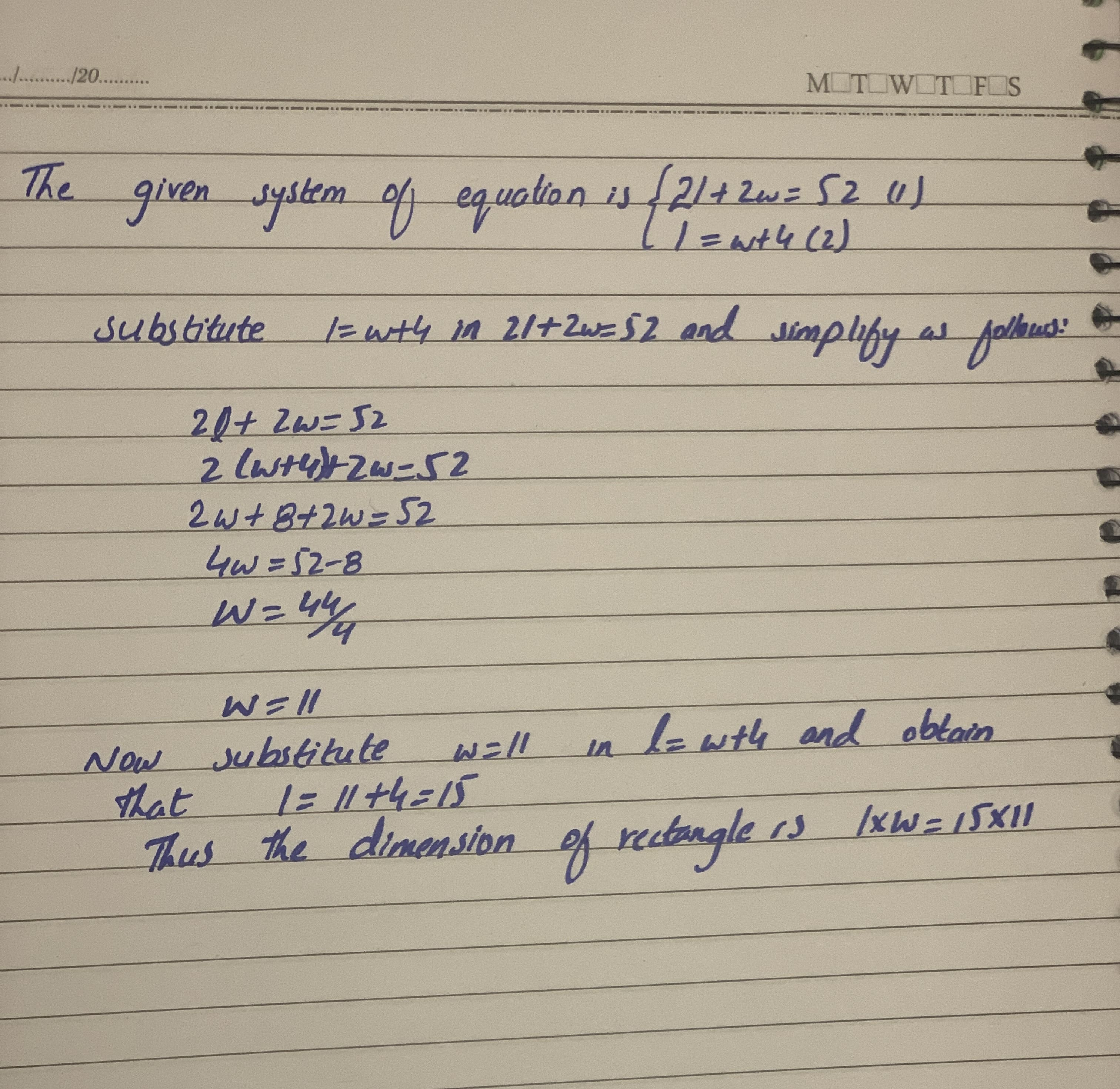Question# Use substitution to solve each system of linear equations in two variables. Dimensions of a rectangle The perimeter of a rectangle is 52 inches.The le

Equations and inequalities
ANSWEREDUse substitution to solve each system of linear equations in two variables.
Dimensions of a rectangle The perimeter of a rectangle is 52 inches.The length of the rectangleis 4 inches more than the width.Determine the dimensions of the rectangle by solving the following system of equations where l and w represent the length and width of the rectangle
$$\begin{cases}2l+2w=52\\l=w+4\end{cases}$$